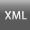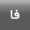Volume 10, Issue 1 (1-2020)2020, 10(1): 31-40 Back to browse issues page
A heuristic method to calculate the real internal rate of return (RIRR)
H. Jafari , M. Faraji, P. Khaleghi Ardehali
Young Researchers and Elite Club, Arak Branch, Islamic Azad Univercity, Arak, Iran
Abstract:   (2339 Views)
The Internal Rate of Return (IRR) is used in economics as a popular method for project evaluation. Its major challenge, however, is to calculate all real rates. This paper presents an algorithm that can be used to calculate all return rates through introducing a heuristic approach based on several mathematical theorems. In order to better understand the different steps of the proposed algorithm, an overall description of the subject was first provided; it was then illustrated with more details through providing some examples. For the purpose of validation and accuracy of the results, the future value of the rates was also calculated at the end of each example. The results of the research showed that the new method had a relatively high efficiency to calculate all real rates. In addition, in the approach proposed in this paper, there is no need for the use of differentiation, and even recurring return rates are also identifiable.
Keywords: Capital Investment Analysis, Cost of Capital, Internal Rate of Return, Present Value